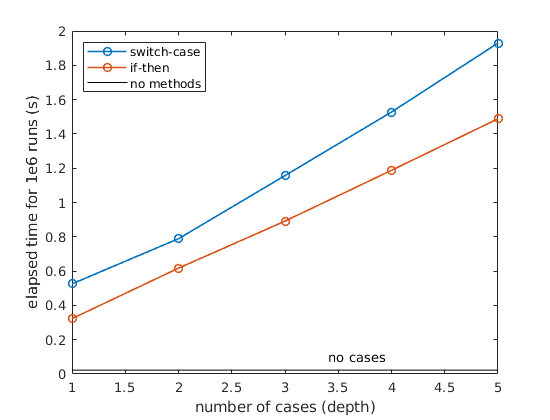# Matlab speed comparison of switch-case and if-then statements and hard-code

I frequently use the extremely methodical approach in scientific programming of “just trying things”. This means that I create a lot of different ways to try to do something to find the best way to do that thing. Sometimes this means getting a realistic result, a stable result, or a fast computation.

All functional programming languages offer if-then statements, whereby sections of code are evaluated on True evaluations of a statement. An if-then statement can be extended with an elseif to provide further evaluations to compare with for True. Using this framework, different “cases” or “methods” of doing a single thing can be quickly tested by switching between them in a script. The purpose of all is to make the code more readable and maintainable, by not having to comment/uncomment blocks of code to make a change in the calculation method.

For example, two methods x = 'test1' | 'test2' could be executed by a function, depending on the definition of x:

A similar functionality can be obtained with a switch-case statement:

switch x
case 'test1'
y = 1;
case 'test2'
y = 2;
end


But which is faster? I suppose I always knew switch-case statements were slower than if-then statements, but I wanted to know how much of a difference it could make. I also often have more than 4 of these case statements as optional methods, and I wanted to know if the number of cases (or how “deep” down the list they were) made a difference in speed.

I designed a simple experiment in Matlab to test this out. I looped through a simple set of statements 1 million times, and timed each scenario. You can find the source code here.It turns out that switch-cases are about ~30% slower than if-then statements. Both are more than an order of magnitude slower than.

Most importantly though, the time increases linearly with each “layer” to the if-then or case-switch statement. To me, this stressed the importance of 1) having few cases that aren’t simply hard-coded in, or 2) at least sort the cases in order of likelihood of being used during program execution.

Updated: